• ## 机器人运动学逆解

千次阅读 2019-11-24 10:44:10
机器人运动学逆解 即根据工具坐标系相对于基坐标系的目标位姿，求解机器人各关节角。逆运动学在机器人学中占有非常重要的地位，是机器人轨迹规划和运动控制的基础，直接影响着控制的快速性与准确性。一般机器人运动...
机器人运动学逆解
即根据工具坐标系相对于基坐标系的目标位姿，求解机器人各关节角。逆运动学在机器人学中占有非常重要的地位，是机器人轨迹规划和运动控制的基础，直接影响着控制的快速性与准确性。一般机器人运动学逆解算法可分为以下几种: 解析法 ( 又称反变换法) 、几 何 法 和 数 值 解法。由 PAUL 等提出的反变换法求解过程直观，因而被广泛采纳，但其求解过程中需多次进行齐次变换矩阵的逆运算和 4 × 4 维矩阵的乘积运算，导致求解过程复杂耗时。


展开全文• 基于MFC的六轴机器人逆解程序，六自由度机器人机械手运动学逆问题反解程序。包含全部源码，可以进行修改，通过更改D-H参数即可实现六轴机器人逆解IK。
• MATLAB机器人求正逆解 手把手教你MATLAB Robotics Toolbox工具箱③ Matlab RoboticToolBox（一）Link参数、三自由度/四自由度逆运动 https://blog.csdn.net/qq_34917736/article/details/89048930
MATLAB机器人求正逆解
手把手教你MATLAB Robotics Toolbox工具箱③
Matlab RoboticToolBox（一）Link参数、三自由度/四自由度逆运动学
https://blog.csdn.net/qq_34917736/article/details/89048930


展开全文matlab
• 为什么要运动学逆解 运动学逆解即根据工具坐标系相对于基坐标系的目标位姿，求解机器人各关节角。 逆运动学在机器人学中占有非常重要的地位，是机器人轨迹规划和运动控制的基础，直接影响着控制的快速性与准确性。...
为什么要运动学逆解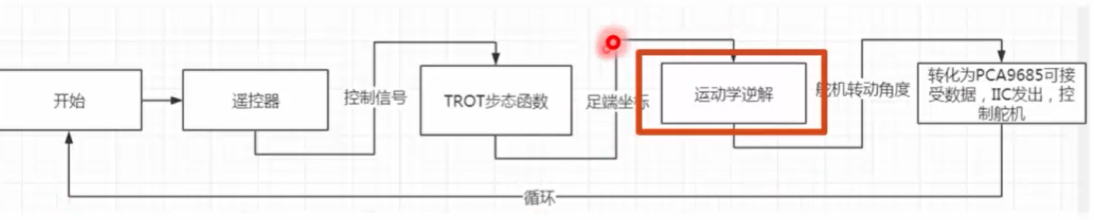运动学逆解即根据工具坐标系相对于基坐标系的目标位姿，求解机器人各关节角。
逆运动学在机器人学中占有非常重要的地位，是机器人轨迹规划和运动控制的基础，直接影响着控制的快速性与准确性。一般机器人运动学逆解算法可分为以下几种: 解析法 ( 又称反变换法) 、几 何 法 和 数 值 解法。由 PAUL 等提出的反变换法求解过程直观，因而被广泛采纳，但其求解过程中需多次进行齐次变换矩阵的逆运算和 4 × 4 维矩阵的乘积运算，导致求解过程复杂耗时。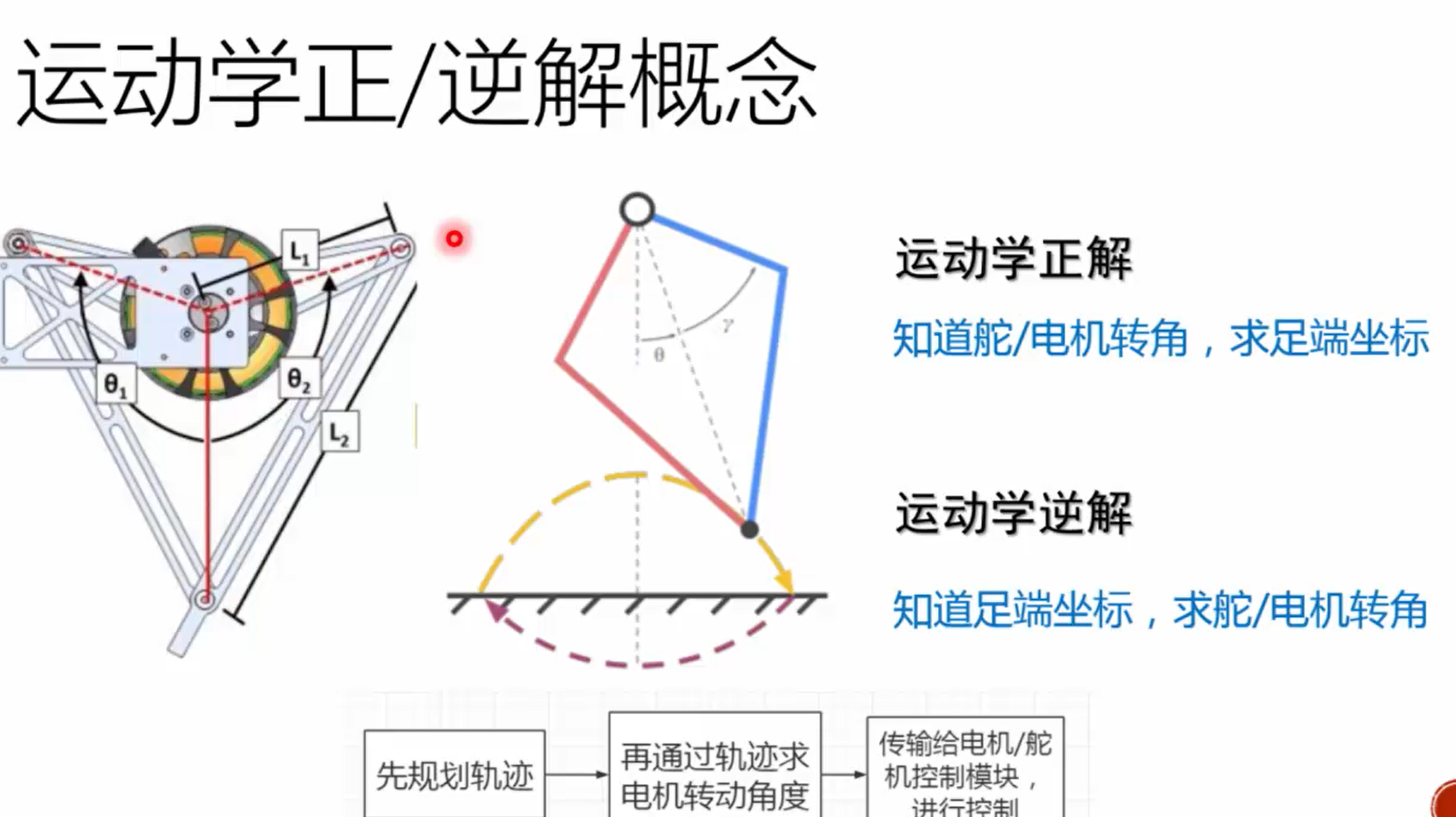足端轨迹规划
在机器人技术各个环节中，信息存在多样性，机器人作业也存在多样性。唯有机器人轨迹规划存在唯一性，机器人的使用都离不开轨迹规划与运动轨迹。随着现在机器人技术的发展，运动规划的原理已慢慢形成共识，对于关节规划逐渐存在主流技术和解决方案。主要会在避障等额外要求下做出规划需求。所以看似不起眼的机器人轨迹规划反而是所有机器人作业中绕不开的一个过程。而且轨迹规划是没有主流要求而言的。按照不同的要求需要不同的轨迹。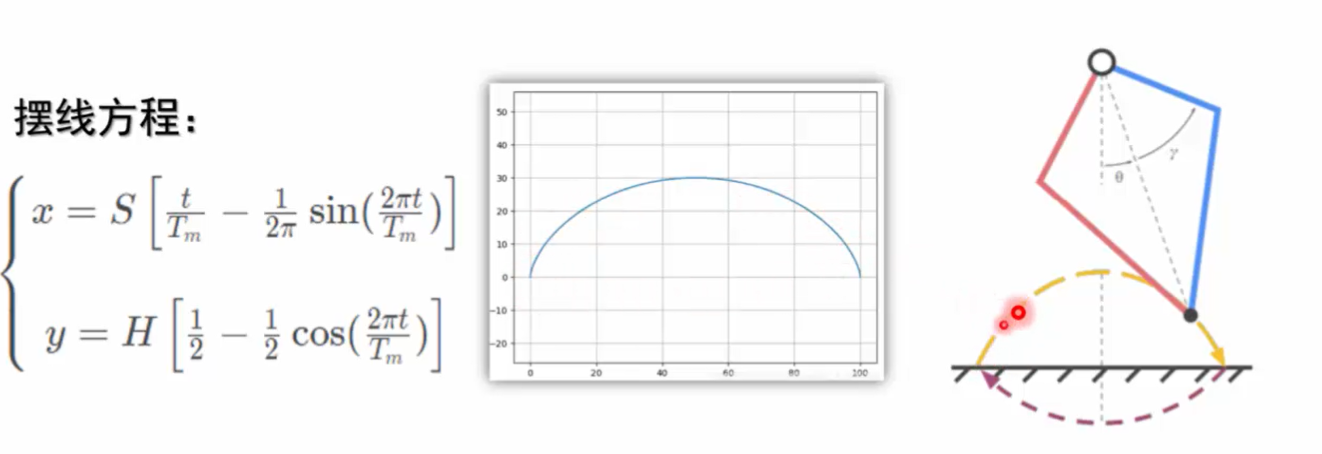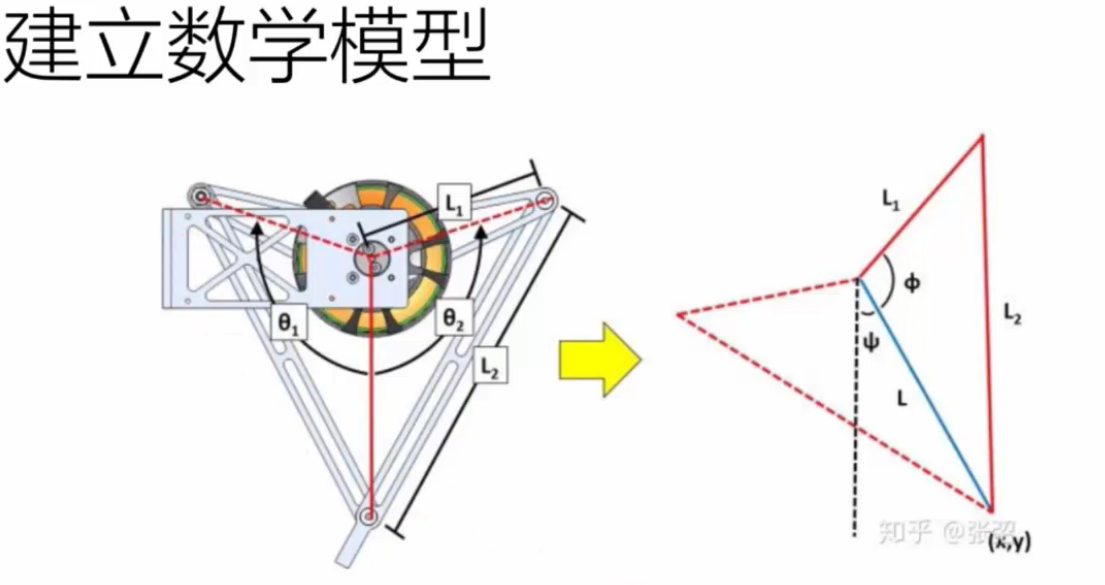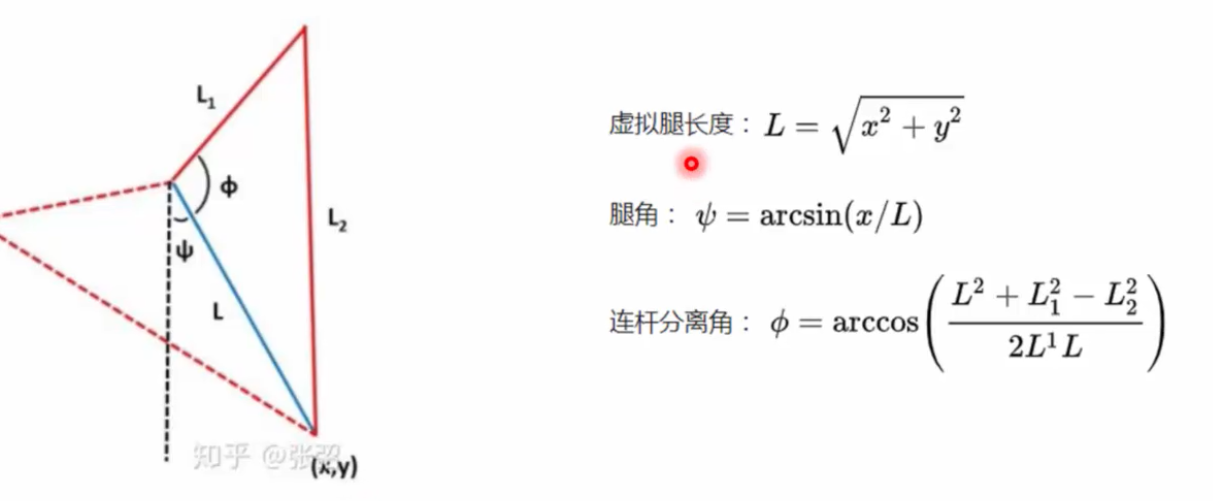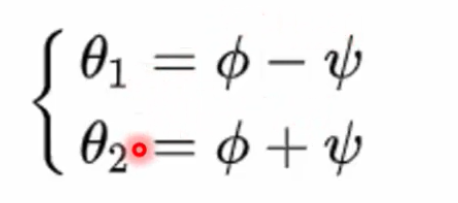课后题求逆解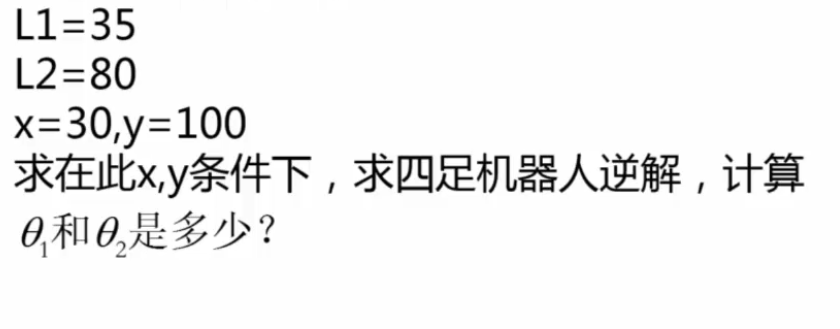解题方法
import math

def fun(x,y,l1,l2):
l=math.sqrt(x*x+y*y)#求虚拟脚长度
psail=math.asin(x/l)#脚角
fail=math.acos((l*l+l1*l1-l2*l2)/(2*l*l1))#连杆分离角
m1=fail-psail#右舵机弧度制
m2=fail+psail#左舵机弧度制
sita1=180*m1/math.pi#右舵机角度制
sita2=180*m2/math.pi#左舵机角度制
return(sita1,sita2)

print(fun(30,100,35,80))

将 L1=35,L2=80,x=30,y=100 输入算法
求得：
右舵机弧度制 0.37927814199991783
左舵机弧度制 0.962191730955652
右舵机角度制 21.731036798158822
左舵机角度制 55.12952526614607
南昌理工学院人工智能学院特种机器人研发中心实验室workshop项目实践平台


展开全文• 基于Matlab三维三轴机器人求逆解（实现简单的定点测试） （机器人建模与仿真中的机器人逆运动和正运动） 其它关于基于Matlab机器人建模与仿真资料合集请往CSDN博客 “基于Matlab的机器人学建模学习资料大整理”...
• 基于改进DH参数的机器人正解和逆解程序，逆解采用解析解形式，输出8组关节角度解
• ## scara机器人运动学正逆解

千次阅读 多人点赞 2020-03-14 14:59:02
文章目录一、scara机器人运动学正解二、scara机器人运动学逆解1、正装scara机器人运动学逆解2、吊装scara机器人运动学逆解三、MATLAB代码 一、scara机器人运动学正解   末端BBB的xxx坐标为向量OA\bf{OA}OA与向量...
文章目录一、scara机器人运动学正解二、scara机器人运动学逆解1、正装scara机器人运动学逆解2、吊装scara机器人运动学逆解3、几个值得思考的问题(1)、手系handcoor的确定(2)、标志位flagJ1与flagJ2的确定(3)、选取最短关节路径逆解三、正逆解正确性验证1、单点验证2、直线验证四、MATLAB代码
一、scara机器人运动学正解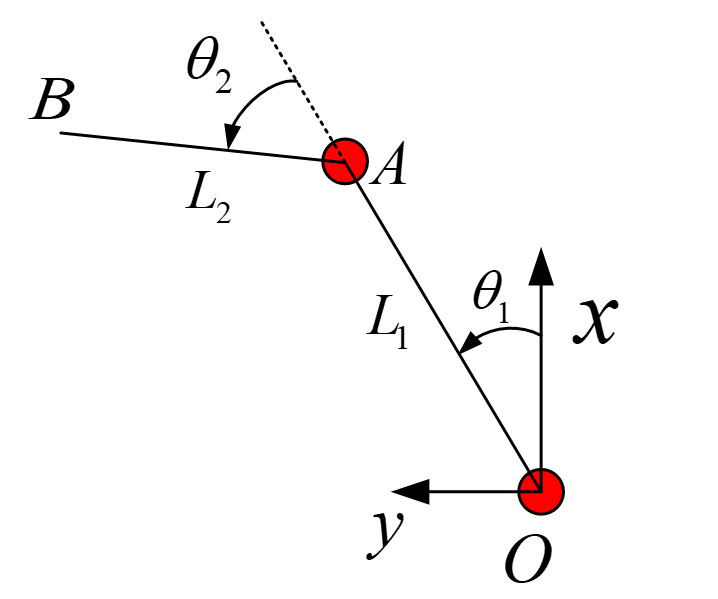末端$B$的$x$坐标为向量$\bf{OA}$与向量$\bf{AB}$在$x$轴上投影之和，末端$B$的$y$坐标亦然：
$\left \{ \begin{array}{c} x=L_1cos\theta_1+L_2cos(\theta_1+\theta_2) \\ \tag 1 y=L_1sin\theta_1+L_2sin(\theta_1+\theta_2) \end{array}\right.$
第三轴为丝杆上下平移运动，设丝杆螺距$s$，末端$B$的$z$坐标：
$z=\frac{\theta_3s}{2\pi} \tag 2$
末端$B$的姿态角$c$：
$c=\theta_1+ \theta_2+ \theta_4 \tag 3$
综上，scara机器人的运动学正解为：
$\begin{cases} x=L_1cos\theta_1+L_2cos(\theta_1+\theta_2) \\ y=L_1sin\theta_1+L_2sin(\theta_1+\theta_2) \\ z=\frac{\theta_3s}{2\pi} \\ c=\theta_1+ \theta_2+ \theta_4 \tag 4 \end{cases}$
二、scara机器人运动学逆解
1、正装scara机器人运动学逆解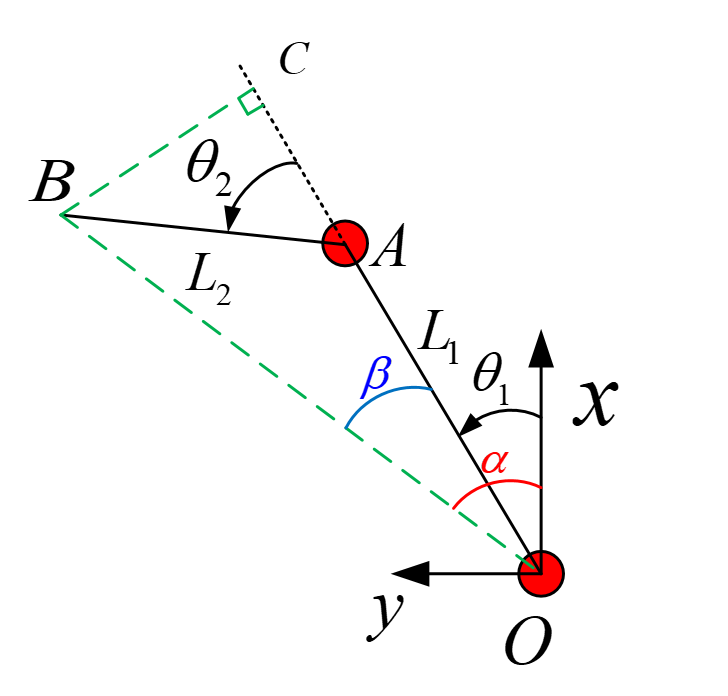连接$OB$，过$B$作$BC$垂直于$OA$于$C$，在$\Delta OAB$中，由余弦定理：
$cos(\pi-\theta_2)=\frac{L_1^2+L_2^2-(x^2+y^2)}{2L_1L_2}\tag 5$
记$c_2=cos\theta_2$，式(5)可写成：
$c_2=\frac{x^2+y^2-L_1^2-L_2^2}{2L_1L_2}\tag 6$
记$s_2=sin\theta_2$，则$s_2$有两个解：
$s_2=\pm\sqrt{1-c_2^2}\tag 7$
取正数解，机器人处于右手系(right handcoor)；取负数解，机器人处于左手系(left handcoor)；特殊地，若$s_2=0$，机器人处于奇异位置(singular position)，此时$\theta_2=k\pi(k\in{Z})$，一般 $\theta_2\in{\{-2\pi,-\pi,0,\pi,2\pi}\}$。
至此，可求得$\theta_2$：
$\theta_2=atan2(s_2,c_2)\tag 8$
如上图，易得：
$\alpha=atan2(y,x)\tag 9$
在$\Delta OBC$中，记$r=\|OB\|$：
$sin\beta=\frac{\|BC\|}{\|OB\|}=\frac{L_2s_2}{r}\tag {10}$
$cos\beta=\frac{\|OC\|}{\|OB\|}=\frac{\|OA\|+\|AC\|}{\|OB\|}=\frac{L_1+L_2c_2}{r}\tag {11}$
由于$r>0$，由式$(10)$和式$(11)$得：
$\beta=atan2(L_2s_2,L_1+L_2c_2)\tag {12}$
至此，可求得$\theta_1$：
$\theta_1=\alpha-\beta=atan2(y,x)-atan2(L_2s_2,L_1+L_2c_2)\tag {13}$
由式$(4)$容易求得$\theta_3$和$\theta_4$。
综上，正装scara机器人的运动学逆解为：
$\begin{cases} \theta_1=atan2(y,x)-atan2(L_2s_2,L_1+L_2c_2) \\ \theta_2=atan2(s_2,c_2) \\ \theta_3=\frac{2\pi z}{s} \\ \theta_4=c-\theta_1-\theta_2 \tag {14} \end{cases}$
在求解$\theta_2$时，完全可以根据式$(6)$用反余弦函数$acos$求得，但这里采用了双变量反正切函数$atan2$，至于原因，可以参见这篇博文：为什么机器人运动学逆解最好采用双变量反正切函数atan2而不用反正/余弦函数？
由于$atan2$的值域为$[-\pi,\pi]$，可得关节角度$\theta_1$在$-2\pi$和$2\pi$之间(区间左端点$-2\pi$和区间右端点$2\pi$不一定能达到)，$\theta_2\in[-\pi,\pi]$，$\theta_3$范围与丝杆螺距$s$和$z$轴的行程有关，$\theta_4$一般范围在$[-2\pi,2\pi]$。对于正装scara机器人，为避免机构干涉，一般$\theta_1\in[-\frac{3\pi}{4},\frac{3\pi}{4}]$，$\theta_2\in[-\frac{2\pi}{3},\frac{2\pi}{3}]$。   式$(14)$的运动学逆解无法做到使机器人在工作空间360°无死角运动，需要将其推广，使得$\theta_1\in[-2\pi,2\pi]$且$\theta_2\in[-2\pi,2\pi]$，即吊装scara机器人关节1和关节2的角度范围。
2、吊装scara机器人运动学逆解
通过式$(14)$计算得到$\theta_1$与$\theta_2$后，增加关节1与关节2角度标志位flagJ1与flagJ2，利用以下的规则，可以将$\theta_1$与$\theta_2$范围限定在$[-2\pi,2\pi]$，实现吊装scara机器人360°无死角运动。
将$\theta_1$范围变换到$[-\pi,\pi]$:
若$\theta_1<-\pi$，则$\theta_1=\theta_1+2\pi$；
若$\theta_1>\pi$，则$\theta_1=\theta_1-2\pi$
对于$\theta_1$，当$flagJ1=1$时：
若$\theta_1\geq0$，则$\theta_1=\theta_1-2\pi$；
若$\theta_1<0$，则$\theta_1=\theta_1+2\pi$
对于$\theta_2$，当$flagJ2=1$时：
若$\theta_2\geq0$，则$\theta_2=\theta_2-2\pi$；
若$\theta_2<0$，$\theta_2=\theta_2+2\pi$
$\theta_4=c-\theta_1-\theta_2$
3、几个值得思考的问题
(1)、手系handcoor的确定
当$\theta_2\in(0,\pi) \bigcup(-2\pi,-\pi)$时，机器人处于右手系，此时$handcoor=1$
当$\theta_2\in(-\pi,0) \bigcup(\pi,2\pi)$时，机器人处于左手系，此时$handcoor=0$
特别的，当$\theta_2\in{\{-2\pi,-\pi,0,\pi,2\pi}\}$，机器人处于奇异位置。
当机器人示教了一个点位，$\theta_2$便确定了，因此机器人当前示教点的手系便确定。
(2)、标志位flagJ1与flagJ2的确定
当$\theta_1\in[-\pi,\pi]$时，$flagJ1=0$；否则，$flagJ1=1$。
当$\theta_2\in[-\pi,\pi]$时，$flagJ2=0$；否则，$flagJ2=1$。
当手系handcoor确定后，便可以根据手系选逆解。当标志位flagJ1与flagJ2确定后，便可以确定关节角度值是否需要$\pm 2\pi$。
(3)、选取最短关节路径逆解
在做笛卡尔空间插补(如直线、圆弧、样条插补等)时，如果$\theta_1\in[-2\pi,2\pi]$且$\theta_2\in[-2\pi,2\pi]$，求逆解过程可能会有$\pm 2\pi$的跳变。这时需要通过对比上一次关节位置，选取最短关节路径的解，以达到插补过程关节位置平滑变化，此时不再需要标志位flagJ1与flagJ2。
三、正逆解正确性验证
1、单点验证
(1)在$[-2\pi, 2\pi]$中随机生成4个关节角度（模拟示教过程）
(2)计算标志位flagJ1和flagJ2，手系handcoor
(3)计算正运动学
(4)计算逆运动学
(5)逆运动学结果与随机生成的关节角度作差，验证运动学正逆解的正确性
2、直线验证
(1)在$[-2\pi,2\pi]$中随机生成4个关节角度，计算手系handcoor（模拟示教过程）
(2)运动学正解计算直线起点坐标(x,y,z,c)
(3)在工作空间里随机获取直线的终点x，y坐标，z在[-50mm,50mm]里随机生成,
c在[-30°, 30°]里随机生成，手系与起点的相同（模拟示教过程）
(4)速度规划(采用归一化五次多项式规划，详见博文：归一化5次多项式速度规划)
(5)直线插补
(6)计算每个插补点的逆运动学
(7)计算每个插补点的正运动学
(8)画出直线插补结果，合成位置、速度、加速度，关节位置曲线，验证正逆解的正确性
四、MATLAB代码
%{
Function: find_handcoor
Description: 计算scara机器人当前手系
Input: 关节2角度theta2(rad)
Output: 机器人手系
Author: Marc Pony(marc_pony@163.com)
%}
function handcoor = find_handcoor( theta2 )
if (theta2 > 0.0 && theta2 < pi) || (theta2 > -2.0 * pi && theta2 < -pi)
handcoor = 1;
else
handcoor = 0;
end
end

%{
Function: find_flagJ1
Description: 计算scara机器人当前J1关节标志位
Input: 关节1角度theta1(rad)
Output: 机器人J1关节标志位flagJ1
Author: Marc Pony(marc_pony@163.com)
%}
function flagJ1 = find_flagJ1( theta1 )
if (theta1 >= -pi && theta1 <= pi)
flagJ1 = 0;
else
flagJ1 = 1;
end
end

%{
Function: find_flagJ2
Description: 计算scara机器人当前J2关节标志位
Input: 关节1角度theta2(rad)
Output: 机器人J2关节标志位flagJ2
Author: Marc Pony(marc_pony@163.com)
%}
function flagJ2 = find_flagJ2( theta2 )
if (theta2 >= -pi && theta2 <= pi)
flagJ2 = 0;
else
flagJ2 = 1;
end
end

%{
Function: scara_forward_kinematics
Description: scara机器人运动学正解
Input: 大臂长L1(mm)，小臂长L2(mm)，丝杆螺距screw(mm)，机器人关节位置jointPos(rad)
Output: 机器人末端位置cartesianPos(mm或rad)
Author: Marc Pony(marc_pony@163.com)
%}
function cartesianPos = scara_forward_kinematics(L1, L2, screw, jointPos)
theta1 = jointPos(1);
theta2 = jointPos(2);
theta3 = jointPos(3);
theta4 = jointPos(4);
x = L1 * cos(theta1) + L2 * cos(theta1 + theta2);
y = L1 * sin(theta1) + L2 * sin(theta1 + theta2);
z = theta3 * screw / (2 * pi);
c = theta1 + theta2 + theta4;
cartesianPos = [x; y; z; c];
end

%{
Function: scara_inverse_kinematics
Description: scara机器人运动学逆解
Input: 大臂长L1(mm)，小臂长L2(mm)，丝杆螺距screw(mm)，机器人末端坐标cartesianPos(mm或rad)
手系handcoor，标志位flagJ1与flagJ2
Output: 机器人关节位置jointPos(rad)
Author: Marc Pony(marc_pony@163.com)
%}
function jointPos = scara_inverse_kinematics(L1, L2, screw, cartesianPos, handcoor, flagJ1, flagJ2)
x = cartesianPos(1);
y = cartesianPos(2);
z = cartesianPos(3);
c = cartesianPos(4);
jointPos = zeros(4, 1);

calculateError = 1.0e-8;
c2 = (x^2 + y^2 - L1^2 -L2^2) / (2.0 * L1 * L2);%若(x,y)在工作空间里，则c2必在[-1,1]里，但由于计算精度，c2的绝对值可能稍微大于1
temp = 1.0 - c2^2;

if temp < 0.0
if temp > -calculateError
temp = 0.0;
else
error('区域不可到达');
end
end
if handcoor == 0    %left handcoor
jointPos(2) = atan2(-sqrt(temp), c2);
else                %right handcoor
jointPos(2) = atan2(sqrt(temp), c2);
end
s2 = sin(jointPos(2));
jointPos(1) = atan2(y, x) - atan2(L2 * s2, L1 + L2 * c2);
jointPos(3) = 2.0 * pi * z / screw;

if jointPos(1) <= -pi
jointPos(1) = jointPos(1) + 2.0*pi;
end
if jointPos(1) >= pi
jointPos(1) = jointPos(1) - 2.0*pi;
end

if flagJ1 == 1
if jointPos(1) >= 0.0
jointPos(1) = jointPos(1) - 2.0*pi;
else
jointPos(1) = jointPos(1) + 2.0*pi;
end
end

if flagJ2 == 1
if jointPos(2) >= 0.0
jointPos(2) = jointPos(2) - 2.0*pi;
else
jointPos(2) = jointPos(2) + 2.0*pi;
end
end
jointPos(4) = c - jointPos(1) - jointPos(2);
end

%{
Function: scara_shortest_path_inverse_kinematics
Description: scara机器人运动学逆解
Input: 大臂长L1(mm)，小臂长L2(mm)，丝杆螺距screw(mm)，机器人末端坐标cartesianPos(mm或rad)
手系handcoor，上一次的关节位置lastJointPos(rad)
Output: 机器人关节位置jointPos(rad)
Author: Marc Pony(marc_pony@163.com)
%}
function jointPos = scara_shortest_path_inverse_kinematics(len1, len2, screw, cartesianPos, handcoor, lastJointPos)
x = cartesianPos(1);
y = cartesianPos(2);
z = cartesianPos(3);
c = cartesianPos(4);
jointPos = zeros(4, 1);

calculateError = 1.0e-8;
c2 = (x^2 + y^2 - len1^2 -len2^2) / (2.0 * len1 * len2);%若(x,y)在工作空间里，则c2必在[-1,1]里，但由于计算精度，c2的绝对值可能稍微大于1
temp = 1.0 - c2^2;

if temp < 0.0
if temp > -calculateError
temp = 0.0;
else
error('区域不可到达');
end
end
if handcoor == 0    %left handcoor
jointPos(2) = atan2(-sqrt(temp), c2);
else                %right handcoor
jointPos(2) = atan2(sqrt(temp), c2);
end
s2 = sin(jointPos(2));
jointPos(1) = atan2(y, x) - atan2(len2 * s2, len1 + len2 * c2);
jointPos(3) = 2.0 * pi * z / screw;

temp = zeros(3, 1);
for i = 1 : 2
temp(1) = abs((jointPos(i) + 2.0*pi) - lastJointPos(i));
temp(2) = abs((jointPos(i) - 2.0*pi) - lastJointPos(i));
temp(3) = abs(jointPos(i) - lastJointPos(i));
if temp(1) < temp(2) && temp(1) < temp(3)
jointPos(i) = jointPos(i) + 2.0*pi;
elseif temp(2) < temp(1) && temp(2) < temp(3)
jointPos(i) = jointPos(i) - 2.0*pi;
else
%do nothing
end
end
jointPos(4) = c - jointPos(1) - jointPos(2);
end

clc;
clear;
close all;

%% 输入参数
len1 = 200.0; %mm
len2 = 200.0; %mm
screw = 20.0; %mm
linearSpeed = 100; %mm/s
linearAcc = 800;   %mm/s^2
orientationSpeed = 200; %°/s
orientationAcc = 600;   %°/s^2
dt = 0.05; %s

%% 单点验证：
%(1)在[-2*pi, 2*pi]中随机生成4个关节角度（模拟示教过程）
%(2)计算标志位flagJ1和flagJ2，手系handcoor
%(3)计算正运动学
%(4)计算逆运动学
%(5)逆运动学结果与随机生成的关节角度作差，验证运动学正逆解的正确性
testCount = 1000000;
res = zeros(testCount, 4);
k = 1;
while k < testCount
theta1 = -2.0*pi + 4.0*pi*rand;
theta2 = -2.0*pi + 4.0*pi*rand;
theta3 = -2.0*pi + 4.0*pi*rand;
theta4 = -2.0*pi + 4.0*pi*rand;
flagJ1 = find_flagJ1( theta1 );
flagJ2 = find_flagJ2( theta2 );
handcoor = find_handcoor( theta2 );
jointPos = [theta1, theta2, theta3, theta4];
cartesianPos = scara_forward_kinematics(len1, len2, screw, jointPos);

jointPos2 = scara_inverse_kinematics(len1, len2, screw, cartesianPos, handcoor, flagJ1, flagJ2);
res(k, :) = [theta1, theta2, theta3, theta4] - jointPos2';
k = k + 1;
end
flag = all(abs(res - 0.0) < 1.0e-8) % flag = [1 1]则表示正逆解正确

while 1
%% 直线验证：
%(1)在[-2*pi, 2*pi]中随机生成4个关节角度，计算手系handcoor（模拟示教过程）
%(2)运动学正解计算直线起点坐标(x,y,z,c)
%(3)在工作空间里随机获取直线的终点x，y坐标，z在[-50mm,50mm]里随机生成,
%   c在[-30°, 30°]里随机生成，手系与起点的相同（模拟示教过程）
%(4)速度规划
%(5)直线插补
%(6)计算每个插补点的逆运动学
%(7)计算每个插补点的正运动学
%(8)画出直线插补结果，合成位置、速度、加速度，关节位置曲线，验证正逆解的正确性
theta1 = -2.0*pi + 4.0*pi*rand;
theta2 = -2.0*pi + 4.0*pi*rand;
theta3 = -2.0*pi + 4.0*pi*rand;
theta4 = -2.0*pi + 4.0*pi*rand;
handcoor = find_handcoor( theta2 );
jointPos = [theta1, theta2, theta3, theta4];
cartesianPos = scara_forward_kinematics(len1, len2, screw, jointPos);
lastJointPos = jointPos;
x1 = cartesianPos(1);
y1 = cartesianPos(2);
z1 = cartesianPos(3);
c1 = cartesianPos(4)*180.0/pi;

figure(1)
set(gcf, 'color','w');
a = 0 : 0.01 : 2*pi;
plot((len1 + len2)*cos(a), (len1 + len2)*sin(a), 'r--')
hold on
plot(x1, y1, 'ko')
xlabel('x/mm');
ylabel('y/mm');
axis equal tight
[x2, y2] = ginput(1);
z2 = -50 + 50*rand;
c2 = -30 + 60*rand;

L = sqrt((x2 - x1)^2 + (y2 - y1)^2 + (z2 - z1)^2);
C = abs(c2 - c1);
T = max([1.875 * L / linearSpeed, 1.875 * C / orientationSpeed, ...
sqrt(5.7735 * L / linearAcc), sqrt(5.7735 * C / orientationAcc)]);

t = (0 : dt : T)';
u = t / T;
if abs(t(end) - T) > 1.0e-8
t = [t; T];
u = [u; 1];
end
u = t / T;
s = 10*u.^3 - 15*u.^4 + 6*u.^5;
ds = 30*u.^2 -60*u.^3 + 30*u.^4;
dds = 60*u - 180*u.^2 + 120*u.^3;

len = L * s;
vel = (L / T) * ds;
acc = (L / T^2) * dds;
pos = zeros(length(t), 4);
jointPos = zeros(length(t), 4);
cartesianPos = zeros(length(t), 4);
rate = [x2 - x1, y2 - y1, z2 - z1, c2 - c1] / L;
for i = 1 : length(t)
pos(i, :) = [x1, y1, z1, c1] + len(i) * rate;
pos(i, 4) = pos(i, 4)*pi/180.0;
jointPos(i, :) = scara_shortest_path_inverse_kinematics(len1, len2, screw, pos(i, :), handcoor, lastJointPos);
cartesianPos(i, :) = scara_forward_kinematics(len1, len2, screw, jointPos(i, :));
lastJointPos = jointPos(i, :);
end
plot3(cartesianPos(:, 1), cartesianPos(:, 2), cartesianPos(:, 3), 'ro');
plot3([x1 x2], [y1 y2], [z1 z2], '+','markerfacecolor','k','markersize', 14)

figure(2)
set(gcf, 'color','w');
subplot(3,1,1)
plot(t, len)
hold on
plot([0 t(end)], [L L], 'r--')
xlabel('t/s');
ylabel('len/mm');
subplot(3,1,2)
plot(t, vel)
hold on
plot([0 t(end)], [linearSpeed linearSpeed], 'r--')
xlabel('t/s');
ylabel('vel/ mm/s');
subplot(3,1,3)
plot(t, acc)
hold on
plot([0 t(end)], [linearAcc linearAcc], 'r--')
plot([0 t(end)], [-linearAcc -linearAcc], 'r--')
xlabel('t/s');
ylabel('acc/ mm/s^2');

figure(3)
set(gcf, 'color','w');
subplot(3,1,1)
plot(t, len*rate(4))
hold on
plot([0 t(end)], [c2-c1 c2-c1], 'r--')
xlabel('t/s');
ylabel('c/°');
subplot(3,1,2)
plot(t, vel*rate(4))
hold on
plot([0 t(end)], [orientationSpeed orientationSpeed], 'r--')
plot([0 t(end)], [-orientationSpeed -orientationSpeed], 'r--')
xlabel('t/s');
ylabel('dc/ °/s');
subplot(3,1,3)
plot(t, acc*rate(4))
hold on
plot([0 t(end)], [orientationAcc orientationAcc], 'r--')
plot([0 t(end)], [-orientationAcc -orientationAcc], 'r--')
xlabel('t/s');
ylabel('ddc/ °/s^2');

figure(4)
set(gcf, 'color','w');
subplot(2,2,1)
plot(t, jointPos(:,1)*180.0/pi)
xlabel('t/s');
ylabel('J1/°');
subplot(2,2,2)
plot(t, jointPos(:,2)*180.0/pi)
xlabel('t/s');
ylabel('J2/°');
subplot(2,2,3)
plot(t, jointPos(:,3)*180.0/pi)
xlabel('t/s');
ylabel('J3/°');
subplot(2,2,4)
plot(t, jointPos(:,4)*180.0/pi)
xlabel('t/s');
ylabel('J4/°');

pause()
close all
end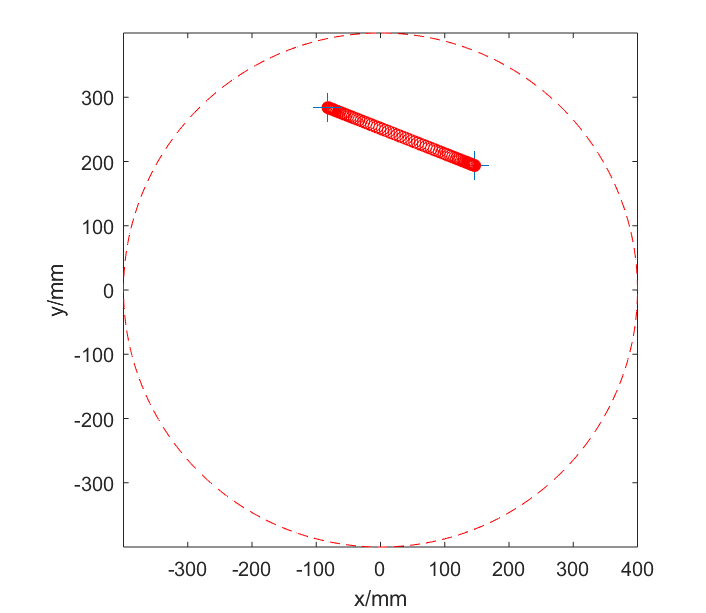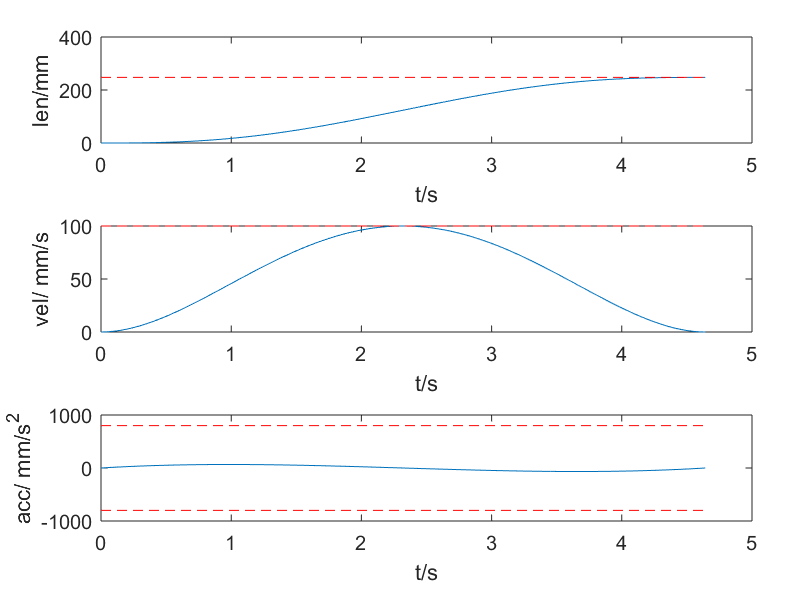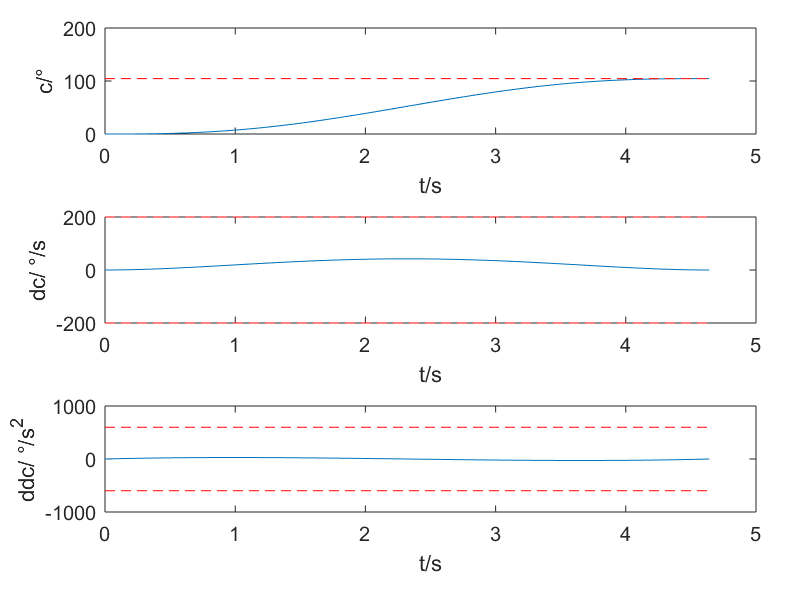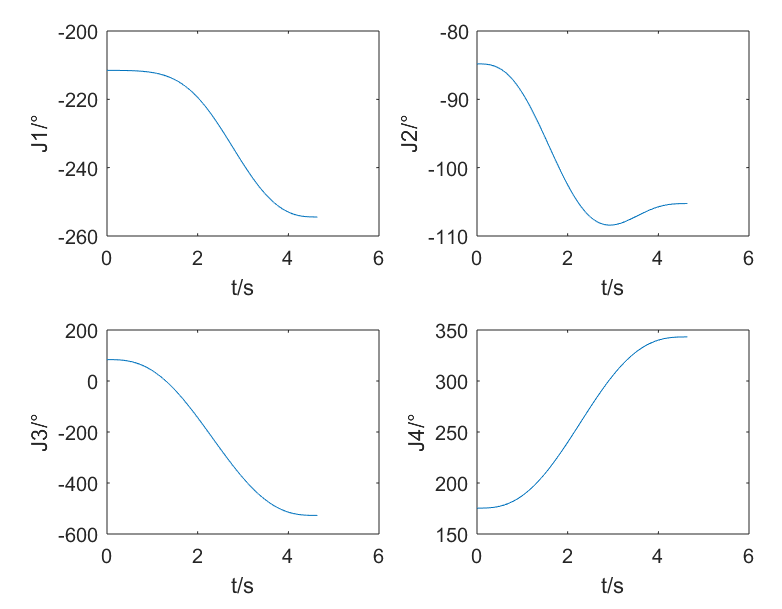展开全文• puma560机器人的正逆解求解以及雅克比矩阵和动力
•研究论文
• 包含了UR机器人的运动建模与运动逆解的求解过程（解析法），通过实际的机器人参数验证该求解方法的正确性，分析了机器人的奇异位置，并编制好matlab程序便与仿真。
• Delta Robot Kinematics 并联机器人 运动 正解 逆解 本人自己总结 matlab亲测 正反解正确
• 机器人基础之运动学逆解概述求解腕点位置求解腕部方位*z-y-z*欧拉角具体求解算例MATLAB实现 概述 运动学逆解是指已知机器人末端位姿，求解各运动关节的位置，它是机器人运动规划和轨迹控制的基础。 以机械臂为例，其...
• 逆解 逆解计算方法可以参考以下书籍 机器人学导论——分析、系统及应用 电子工业出版社 机器人学导论第3版 机械工业出版社 机器人学建模、规划与控制 西安交通大学出版社 对于关节1,2,3可以从运动方程手工推导出...
•   今天，讲讲机器人运动学逆解中的一大“法宝”，最常用的三角方程： k1sin(θ)+k2cos(θ)=k3(1) k_1sin(\theta)+k_2cos(\theta)=k_3 \tag{1} k1​sin(θ)+k2​cos(θ)=k3​(1)   联立三角恒等式： sin2(θ)+cos...
• 并联机器人由多个封闭的机构环组成。这些机构环通常是由连接基座和...本文根据DELTA机器人的机构结构，运用空间向量知识，建立机器人各连杆之间位置的向量关系，进行DELTA机器人的运动学逆解计算，以及工作空间的计算。
• ## 6轴机器人运动学正解,逆解1

万次阅读 多人点赞 2017-12-14 21:27:35
给定机器人各关节的角度，计算出机器人末端的空间位置逆解 已知机器人末端的位置和姿态，计算机器人各关节的角度值常见的工业机器人 正解与逆解的求解需要相应的机器人运动方程，其中关键的就是DH参数表 DH参数...DH参数
• 其中运动学逆解直接输出给舵机，控制机器人的运动，因此运动学逆解很重要。 2 基本概念 2.1机械结构模型   对于8自由度机器人，其机械结构模型如下图所示。图中两个并联大腿L1L_1L1​杆分别由独立的舵机带动，假设...
• 用于matlab中的神经网络开发 rbf神经网络求解机器人的运动学逆解源码
• 2.运动学逆解:已知足端坐标，求舵机/电机转角。 二.足端轨迹规划 摆线方程: { x=r*(t-sint) y=r*(1-cost) [其中r为圆的半径，t是圆的半径所经过的弧度（滚动角），当t由0变到2π时，动点就画出了摆线的一支，称为一...
• 最近求机械臂的逆解的解析解，出现了一个三角函数式，虽然用笨方法解出来了，可是有点太浪费时间,于是找到了一种高中常用的方法，方程如下： acosθ+bsinθ=ca cos\theta +bsin\theta =cacosθ+bsinθ=c 解法：tan...
• 六自由度机器人机械手运动学逆问题反程序。。。。
• 运用回转变换张量法。求解了三个腕关节轴线相交于一点的6自由度喷涂机器人的运动学逆解， 并利用消元法简化了运动学逆解的求解过程。得出了较为简易的解析解。
• 根据角度计算坐标为运动鞋正解，而逆解便是反过来根据坐标求角度便是运动学逆解 2.1正解 根据弧度求解坐标方程 2.2逆解 根据坐标和已知边长度求解角度 （注：这里面的θ是以垂直边即上图的那个垂直边开始算而不是...人工智能
• 机器人运动是正运动过程，即已知机器人末端执行器在参考坐标系中的位置和姿态，求解机器人各关节运动变量的过程。机器人逆运动机器人作业运动规划和轨迹控制的基础，求解运动的过程也比求解正......